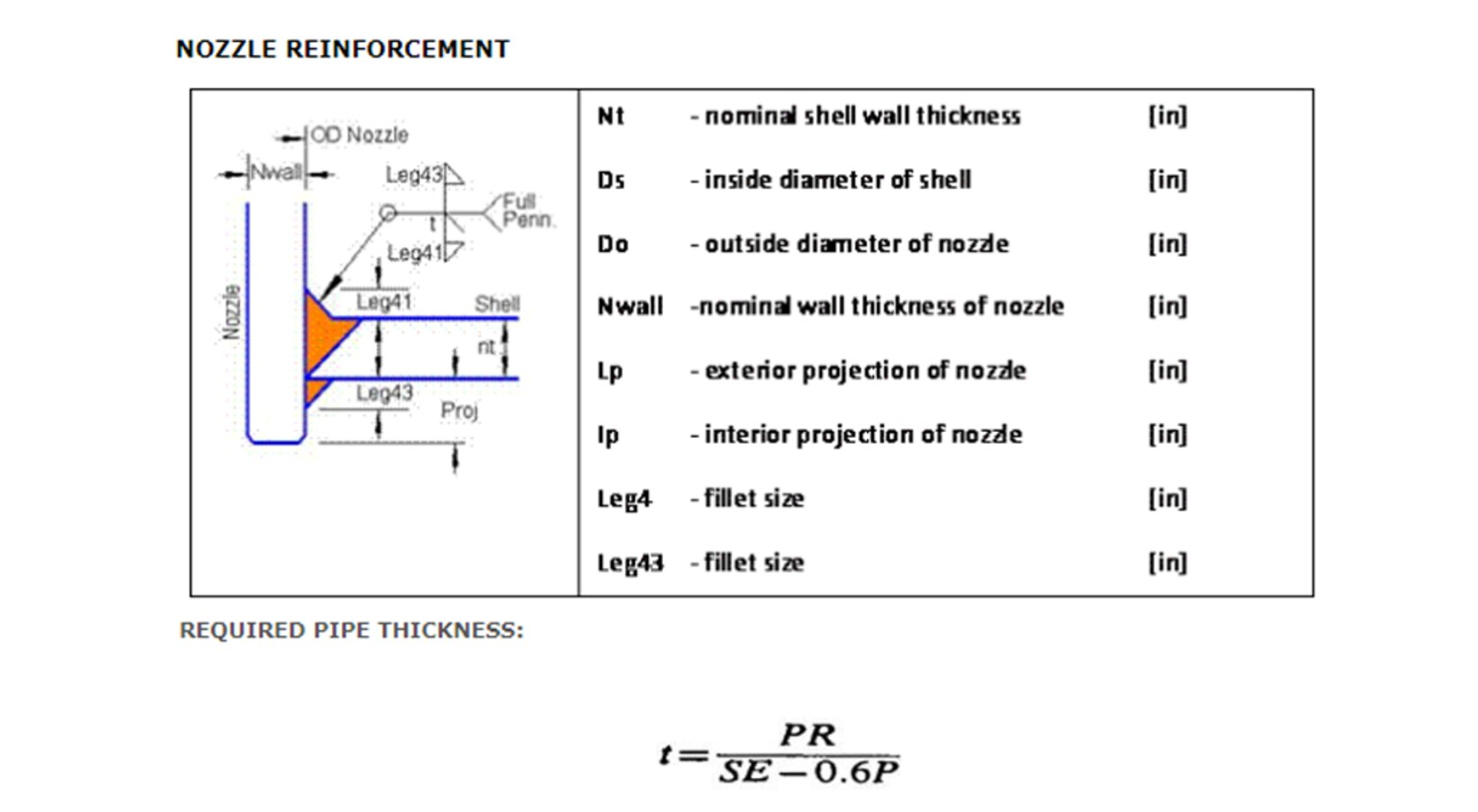NozzleWhere,
P = Internal pressure in psi
R = Nozzle inside diameter in inches = (Outer Radius/2) - (Nwall-nca) + UT
Nca = nozzle corrosion allowance in inches
UT = under tollerence in inches
S = allowable stress in psi
E = joint efficiency
Taken thickness value for the corresponding nominal thickness from nozzle table should be greater than to calculated thickness value from formula shown above.
AREA REPLACEMENT CALCULATION (WITH OUT RF PAD) : (FROM ASME FIG UG-37.1)
Area required (A)
A = d tr F+2 tn tr F (1-fr1)
Where,
d = Finished opening diameter in inches = (Do-2) * tn
tr = required pipe thickness in inches
F = correction factor
tn = nozzle wall thickness in inches
fr1 = Sn/Sv for nozzle wall inserted through the vessel wall
Sn = allowable stress in nozzle in psi
Sv = allowable stress in vessel in psi
Actual area
Actual Area (Aa) = (A1 + A2 + A3 + A41 + A43)
Where,
* Area available in shell (A1):
Area available in shell is equal to the maximum value which may either be A11 or A12.
A11 = d (E*t- F tr) - 2tn (E*t- F tr)*(1-fr1) (in2)
A12 = 2(t + tn ) * (E*t- F tr) - 2tn (E*t- F tr)*(1-fr1) (in2)
* Area available in nozzle projecting outward (A2):
Area available in nozzle projecting outward is equal to the minimum value which may either be A21 or A22
A21 = 5(tn - trn) fr2t (in2)
A22 = 5(tn - trn) fr2tn (in2)
* Area available in inward nozzle (A3):
Area available in inward nozzle is equal to the minimum value which may either be A31 or A32 or A33
A31 = 5*t*ti*fr2 (in2)
A32 = 5* ti *ti*fr2 (in2)
A33 = 5* h *ti*fr2 (in2)
* Area available in outward and inward welds (A41 & A43):
WELD
If (A1 + A2 + A3 + A41 + A43) >= A, Opening is adequately reinforced
If (A1 + A2 + A3 + A41 + A43) < A, Opening is not adequately reinforced so reinforcing elements must be added and/or thicknesses must be increased
Where,
A1 = Area available in shell (in2)
A2 = Area available in nozzle projecting outward (in2)
A3 = Area available in inward nozzle (in2)
A41 = Area available in outward weld (in2)
A43 = Area available in inward weld (in2)
Aa = Actual Area (in2) = (A1 + A2 + A3 + A41 + A43)
AREA REPLACEMENT CALCULATION (WITH RF PAD):(FROM ASME FIG UG-37.1)
Area required (A)
A = d tr F+2 tn tr F (1-fr1)
Where,
d = Finished opening diameter in inches = (Do-2) * tn
tr = required pipe thickness in inches
F = correction factor
tn = nozzle wall thickness in inches
fr1 = Sn/Sv for nozzle wall inserted through the vessel wall
Sn = allowable stress in nozzle in psi
Sv = allowable stress in vessel in psi
Actual area
Actual Area (Aa) = (A1 + A2 + A3 + A41 + A42 + A43 + A5)
Where,
* Area available in shell (A1):
Area available in shell is is equal to the maximum value which may either be A11 or A12.
A11 = d (E*t- F tr) - 2tn (E*t- F tr)*(1-fr1) (in2)
A12 = 2(t + tn ) * (E*t- F tr) - 2tn (E*t- F tr)*(1-fr1) (in2)
* Area available in nozzle projecting outward (A2):
Area available in nozzle projecting outward is equal to the minimum value which may either be A21 or A22
A21 = 5(tn - trn) fr2t (in2)
A22 = 2(tn - trn) * (2.5tn + te) * fr2 (in2)
* Area available in inward nozzle (A3):
Area available in inward nozzle is equal to the minimum value which may either be A31 or A32 or A33
A31 = 5*t*ti*fr2 (in2)
A32 = 5* ti *ti*fr2 (in2)
A33 = 5* h *ti*fr2 (in2)
1111
* Area available in outward and inward welds (A41 & A43):
If (A1 + A2 + A3 + A41 + A43) >= A, Opening is adequately reinforced
If (A1 + A2 + A3 + A41 + A43) < A, Opening is not adequately reinforced so reinforcing elements must be added and/or thicknesses must be increased
So the required area of nozzzle on shell should be less than to the available area for nozzle on shell.
A1 = Area available in shell (in2)
A2 = Area available in nozzle projecting outward (in2)
A3 = Area available in inward nozzle (in2)
A41 = Area available in outward weld (in2)
A43 = Area available in inward weld (in2)
Aa = Actual Area (in2) = (A1 + A2 + A3 + A41 + A43)
d = Finished opening diameter in inches = (Do-2) * tn
t = specified vessel wall thickness in inches (not including forming allowances and under tolerance)
tr = required pipe thickness in inches
tn = nozzle wall thickness in inches
trn = required thickness of a seamless nozzle wall in inches
te = thickness or height of reinforcing element in inches
ti = nominal thickness of internal projection of nozzle wall in inches
Sn = allowable stress in nozzle in psi
Sp = allowable stress in reinforcing element (plate) in psi
Sv = allowable stress in vessel in psi
F = correction factor
fr1 = 1.0 for nozzle wall abutting the vessel wall OR Sn/Sv for nozzle wall inserted through the vessel wall
fr2 = ratio of Sn/Sv
fr3 = lesser value of (Sn/Sv, Sp/Sv)
fr3 = ratio of Sp/Sv
h = distance nozzle projects beyond the inner surface of the vessel wall